## The ASTC algorithm

This section of the guide explains how the ASTC algorithm works. We start with a high-level overview of the block compression algorithm and color encoding process, then we examine the technical details.

### Block compression

Compression formats for real-time graphics need the ability to quickly and efficiently make random samples into a texture. This places two technical requirements on any compression format. It must be possible to do the following:

• Compute the address of data in memory given only a sample coordinate.
• Decompress random samples without decompressing too much surrounding data.

The standard solution that all contemporary real-time formats use, including ASTC, is to divide the image into fixed-size blocks of texels. Each block is then compressed into a fixed number of output bits. This feature makes it possible to access texels quickly, in any order, and with a well-bounded decompression cost.

The 2D block footprints in ASTC range from 4x4 texels up to 12x12 texels, which all compress into 128-bit output blocks. By dividing 128 bits by the number of texels in the footprint, we derive the format bit rates. These bit rates range from 8 bpt (128/(4*4)) down to 0.89 bpt (128/(12*12)).

### Color encoding

ASTC uses gradients to assign the color values of each texel. Each compressed block stores the end-point colors for a gradient, and an interpolation weight for each texel. During decompression, the color value for each texel is generated by interpolating between the two end-point colors, based on the per-texel weight. The following diagram shows this interpolation for a variety of texel weights: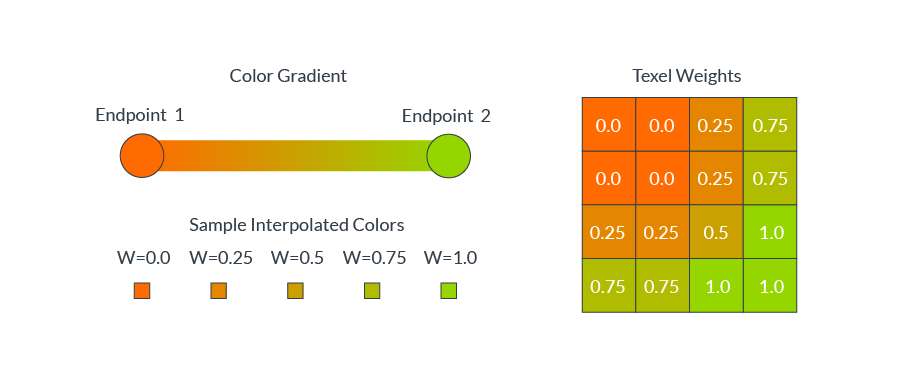Blocks often contain a complex distribution of colors, for example a red ball sitting on green grass. In these scenarios, a single-color gradient cannot accurately represent all different texel color values. ASTC allows a block to define up to four distinct color gradients, called partitions, and can assign each texel to a single partition. For our example, we require two partitions, one for the red ball texels and one for the green grass texels. The following diagram shows how the partition index specifies which color gradient to use for each texel: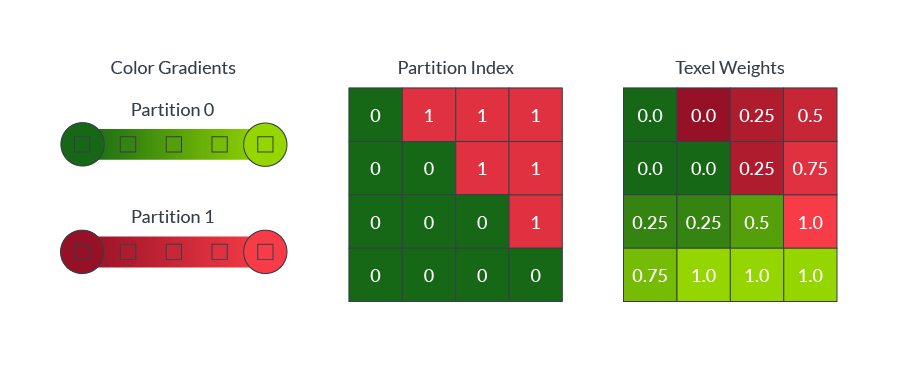### Storing alphabets

Even though color and weight values per texel are notionally floating-point values, we have too few bits available to directly store the actual values. To reduce the storage size, these values must be quantized during compression. For example, if we have a floating-point weight for each texel in the range 0.0 to 1.0, we could choose to quantize to five values: 0.0, 0.25, 0.5, 0.75, and 1.0. We can then represent these five quantized values in storage using the integer values 0-4.

In the general case, if we choose to quantize N levels, we need to be able to efficiently store characters of an alphabet containing N symbols. An N symbol alphabet contains log2(N) bits of information per character. If we have an alphabet of five possible symbols, then each character contains ~2.32 bits of information, but simple binary storage would require us to round up to three bits. This wastes 22.3% of our storage capacity.

The following chart shows the percentage of the bit-space that would be wasted using simple binary encoding to store an arbitrary N symbol alphabet: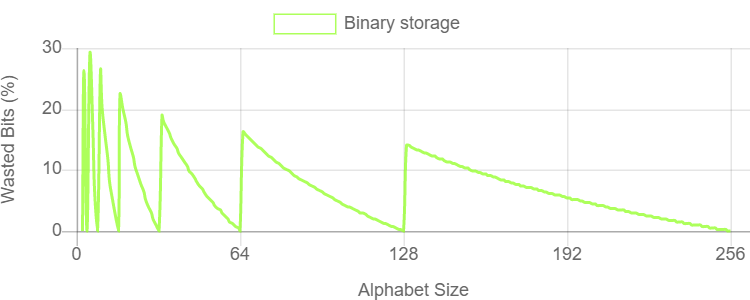This chart shows that for most alphabet sizes, using an integer number of bits per character wastes a lot of storage capacity. Efficiency is critically important to a compression format, so this is an area that ASTC needed to address.

One solution is to round the quantization level up to the next power of two, so that rather than being wasted the extra bits are used. However, this solution forces the encoder to spend bits which could be used elsewhere for a bigger benefit, so it reduces image quality and is a suboptimal solution.

### Quints and trits

Instead of rounding up a five-symbol alphabet, called a quint, to three bits, a more efficient solution is to pack three quint characters together. Three characters in a five-symbol alphabet have 5^3 (125) combinations, and contain 6.97 bits of information. We can store these three quint characters in seven bits and have a storage waste of only 0.5%.

We can similarly construct a three-symbol alphabet, called a trit, and pack trit characters in groups of five. Each character group has 3^5 (243) combinations, and contains 7.92 bits of information. We can store these five trit characters in eight bits and have a storage waste of only 1%.

### Bounded Integer Sequence Encoding

The Bounded Integer Sequence Encoding (BISE), that ASTC uses, allows storage of character sequences using arbitrary alphabets of up to 256 symbols. Each alphabet size is encoded in the most space-efficient choice of bits, trits, and quints.

• Alphabets with up to (2^n - 1) symbols can be encoded using n bits per character.
• Alphabets with up 3x(2^n - 1) symbols can be encoded using n bits (m) and a trit (t) per character, and reconstructed using the equation ((t x 2^n) + m).
• Alphabets with up to 5x(2^n - 1) symbols can be encoded using n bits (m) and a quint (q) per character, and reconstructed using the equation ((q x 2^n) + m).

When the number of characters in a sequence is not a multiple of three or five we must avoid wasting storage at the end of the sequence, so we add another constraint on the encoding. If the last few values in the sequence to encode are zero, the last few bits in the encoded bit string must also be zero. Ideally, the number of nonzero bits is easily calculated and does not depend on the magnitudes of the previous encoded values. This is challenging to arrange during compression, but it is possible. This means that we do not need to store any padding after the end of the bit sequence, because we can safely assume that they are zero bits.

With this constraint in place, and by some smart packing of the bits, trits, and quints, BISE encodes a string of S characters in an N symbol alphabet using a fixed number of bits:

• S values up to (2^N - 1) use (NxS) bits.
• S values up to (3 * 2^N - 1) use (NxS + ceil(8S / 5)) bits.
• S values up to (5 * 2^N - 1) use (NxS + ceil(7S / 3)) bits.

The compressor chooses the option which produces the smallest storage for the alphabet size that is being stored. Some use binary, some use bits and a trit, and some use bits and a quint. If we compare the storage efficiency of BISE against simple binary for the range of possible alphabet sizes that we might want to encode, we see that BISE is much more efficient. The following chart shows the efficiency gain of BISE storage over binary storage: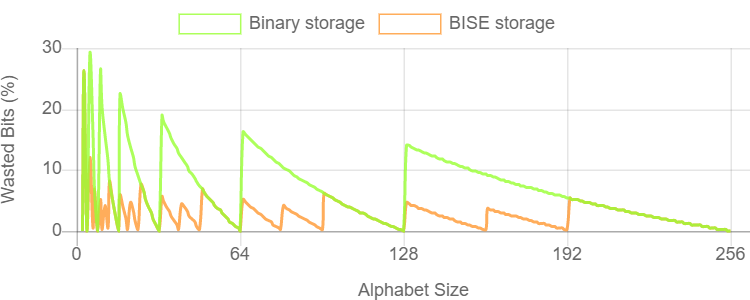### Block sizes

ASTC always compresses blocks of texels into 128-bit outputs. However, ASTC allows the developer to select from a range of block sizes to enable a fine-grained tradeoff between image quality and size. The following table shows the different block sizes result and the corresponding bits per texel:

Block footprint Bits per texel
4x4 8.00
5x4 6.40
5x5 5.12
6x5 4.27
6x6 3.56
8x5 3.20
8x6 2.67
10x5 2.56
10x6 2.13
10x8 1.60
10x10 1.28
12x10 1.07
12x12 0.89

### Color endpoints

The color data for a block is encoded as a gradient between two color endpoints. Each texel selects a position along that gradient, which is then interpolated during decompression. ASTC supports 16 color endpoint encoding schemes, known as endpoint modes.

The options for endpoint modes let you vary the following:

• The number of color channels. For example, luminance, luminance+alpha, rgb, or rgba
• The encoding method. For example, direct, base+offset, base+scale, or quantization level
• The data range. For example, low dynamic range or High Dynamic Range

The endpoint modes, and the endpoint color BISE quantization level, can be chosen on a per-block basis.

### Color partitions

Colors within a block are often complex. A single-color gradient often cannot accurately capture all the colors within a block. For example, the red ball lying on green grass described earlier in this section requires two color partitions, as shown in the following diagram:ASTC allows a single block to reference up to four color gradients, called partitions. Each texel is then assigned to a single partition for the purposes of decompression.

Directly storing the partition assignment for each texel would need a lot of decompressor hardware to store it for all block sizes. Instead, ASTC algorithmically generates a range of patterns, using the partition index as a seed value. The compression process selects the best pattern match for each block. The block then only needs to store the index of the best matching pattern. The following image shows the generated patterns for two (top section of image), three (middle section of image), and four (bottom section of image) partitions for the 8x8 block size: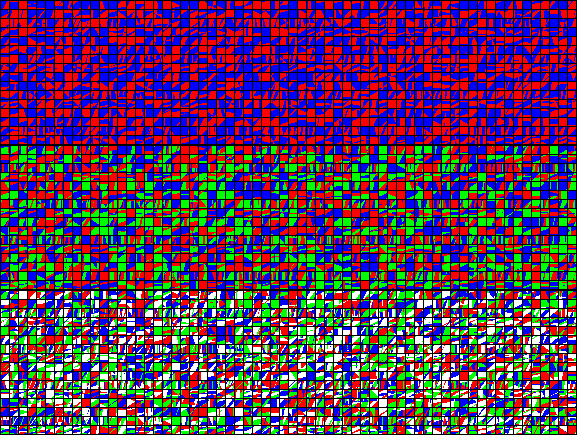The number of partitions and the partition index can be chosen on a per-block basis, and a different color endpoint mode can be chosen per partition.

### Choosing the best encoding for each block

During compression, the algorithm must select the correct distribution pattern and boundary color pairs, then generate the quantized values for each pixel. There is a certain degree of trial and error involved in the selection of patterns and boundary colors, so when compressing there is a trade-off between compression time and final image quality. The higher the quality, the more alternatives the algorithm tries before deciding which is best. However long the compression takes, the decompression time is fixed. This is because the image data can always be re-extrapolated from the pattern and boundary colors in a single pass.

The compression algorithm can use different metrics to judge the quality of different attempts. These metrics range from pure value ratios of signal to noise, to a perceptual judgment weighted towards human visual acuity. The algorithm can also judge the channels individually rather than as a whole. Treating channels individually preserves detail for textures where the individual channels may be used as a data source for a shader program, or to reduce angular noise, which is important for tangent space normal maps.

### Texel weights

Each texel requires a weight, which defines the relative contribution of each color endpoint when interpolating the color gradient.

For smaller block sizes, we can choose to store the weight directly, with one weight per texel. However, for the larger block sizes there are not enough bits of storage to do this. To work around this issue, ASTC allows the weight grid to be stored at a lower resolution than the texel grid. The per-texel weights are interpolated from the stored weight grid during decompression using a bilinear interpolation.

Both the number of texel weights, and the weight value BISE quantization level, can be chosen on a per-block basis.

### Dual-plane weights

Using a single weight for all color channels works well when there is good correlation across the channels, but this is not always the case. Common examples where we might get low correlation include:

• Textures storing RGBA data. Alpha masks are not usually closely correlated with the color value.
• Textures storing normal data. The X and Y normal values often change independently.

ASTC provides a dual-plane mode, which uses two separate weight grids for each texel. A single channel can be assigned to a second plane of weights, while the other three use the first plane of weights.

The use of dual-plane mode can be chosen on a per-block basis, but its use prevents the use of four-color partitions. This is because there are not enough bits to concurrently store both an extra plane of weights and an extra set of color endpoints.